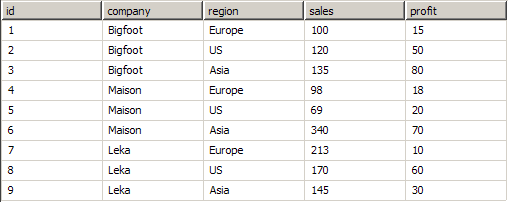﻿ Sorting
Nevron Vision for SharePoint Documentation
Sorting

The examples in this topic consider the sample data set described in Grouping, Filtering and Sorting:Sorting rules can be assigned to each pivot data grouping.

Like filtering, sorting is performed in two modes - group sorting and records sorting. The pivot engine automatically chooses between them depending on the presence/absence of data grouping expressions. In both cases the sorting order is defined by a set of sorting rules.

Sorting Rules

A sorting rule is defined by an Expression and Direction pair. The Direction can be Ascending or Descending. For each pair of input elements (groups or records) E1 and E2 the sorting rules define their order (i.e. E1 < E2, E1 = E2 or E1 > E2) by evaluating the first sorting rule expression towards E1 and E2 and comparing the resulting values in the context of the sorting direction. If the values are equal the operation is performed for the next sorting rule and so on. The elements are sorted given this order.

Group Sorting

Group sorting is performed when the data grouping has grouping expressions. The goal of group sorting is to order the filtered groups for which data members should be created. For example:

Let A be a root data grouping with a single grouping expression:=Fields!company and a single sorting rule: Expression:=SUM(Fields!sales), Direction:Descending. The generated groups are: A1={1,2,3}, A2={4,5,6} and A3={7,8,9}. The sum of sales for A1 is (100+120+135)=355, for A2 the sum of sales is (98+69+340)=507, for A3 the sum of sales is (213+170+145)=528. Hence the data members order is AM3, AM2, AM1 (descending).

Record Sorting

Record sorting is performed when the data grouping does not have grouping expressions. The goal of record sorting is to order the filtered records, for which a data member should be created. It makes no sense to use aggregate functions in record filter expressions, since they are always evaluated towards a single record from the input recordset. For example:

Let A be a root data grouping with no grouping expressions and a single sorting rule: Expression:=Fields!sales, Direction:Ascending. Also let A has two properties - P1 with expression:=FIRST(Fields!sales) and P2 with expression:=LAST(Fields!sales). The input recordset is {1,2,3,4,5,6,7,8,9} while the group recordset will be {5,4,1,2,3,9,8,7,6) (sorted by sales in ascending order) - hence the P1 value will be 69 and the P2 value will be 340.